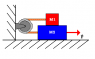# Acceleration of the lower block

• brunettegurl

## Homework Statement

In the diagram shown below, the lower block is acted on by a force, F, which has a magnitude of 58.6 N. The coefficient of kinetic friction between the lower block and the surface is 0.204. The coefficient of kinetic friction between the lower block and the upper block is also 0.204. What is the acceleration of the lower block, if the mass of the lower block is 4.68 kg and the mass of the upper block is 2.11 kg?

## Homework Equations

F=ma / friction = $$\mu$$mg

## The Attempt at a Solution

so i wrote the forces that affect each box

For the upper box ::$$\sum$$ F= T-$$\mu$$m1g
for the lower box :: $$\sum$$ F= F-T- friction1- friction2

i solved the first equation for tension and then substituted it into the 2nd equation and i solved for acceleration...can u pls. tell me what I'm doing wrong thanks#### Attachments

•kn-pic0828.png
1.2 KB · Views: 715
so i wrote the forces that affect each box

For the upper box ::$$\sum$$ F= T-$$\mu$$m1g
for the lower box :: $$\sum$$ F= F-T- friction1- friction2
OK, but what did you use for the friction forces on the lower box?
i solved the first equation for tension and then substituted it into the 2nd equation and i solved for acceleration...can u pls. tell me what I'm doing wrong thanksI assume you applied Newton's 2nd law to each box to get two equations. Show exactly what you did and then we can see what went wrong.

for friction 1 i used (coeffecient of frictionxmass1xgravity) and for friction 2 i use (coeffecient of frictionxmass2xgravity)
and the equation i got before isolating for acceleration is ::

a(m2+m1)= Fapplied-(coeffecient of frictionxmass1xgravity)-(coeffecient of frictionxmass1xgravity)-(coeffecient of frictionxmass2xgravity)

so then a = [Fapplied-(coeffecient of frictionxmass1xgravity)-(coeffecient of frictionxmass1xgravity)-(coeffecient of frictionxmass2xgravity)] /(m1+m2)

for friction 1 i used (coeffecient of frictionxmass1xgravity)
OK.
and for friction 2 i use (coeffecient of frictionxmass2xgravity)
Careful: What's the normal force between the bottom block and the floor?

is it f 2 = coeffecient of frictionxmass2+ mass1xgravity...?

is it f 2 = coeffecient of frictionxmass2+ mass1xgravity...?
Yes, the normal force is the combined weight of both blocks, thus f2 = μ(m1 + m2)g.

ok so i changed that and my answer is still coming out wrong is there anything else wrong i cld have done in the initial equation??

ok so i changed that and my answer is still coming out wrong is there anything else wrong i cld have done in the initial equation??

my final equation wld look like ::
m2a = [Fapplied-m1a-(coeffecient of frictionxmass1xgravity)-(coeffecient of frictionxmass1=mass2xgravity)-(coeffecient of frictionxmass2xgravity)]

a = [Fapplied-(coeffecient of frictionxmass1xgravity)-(coeffecient of frictionxmass1=mass2xgravity)-(coeffecient of frictionxmass2xgravity)] /(m1+m2)

Fapplied is the force given in the question

my final equation wld look like ::
m2a = [Fapplied-m1a-(coeffecient of frictionxmass1xgravity)-(coeffecient of frictionxmass1=mass2xgravity)-(coeffecient of frictionxmass2xgravity)]

a = [Fapplied-(coeffecient of frictionxmass1xgravity)-(coeffecient of frictionxmass1=mass2xgravity)-(coeffecient of frictionxmass2xgravity)] /(m1+m2)

Fapplied is the force given in the question
Your equations are a bit confusing, due to what may be a few typos. (The '=', for one.) Can you rewrite using F, m1, m2, μ, and g? Try to simplify as much as possible.

m2a= [F -m1a- (μm1g)- (μg(m1+m2)) - (μm2g)]

a = [F - (μm1g) - (μg(m1+m2)) - (μm2g)]/(m1+m2)

iss that clearer ??

m2a= [F -m1a- (μm1g)- (μg(m1+m2)) - (μm2g)]

a = [F - (μm1g) - (μg(m1+m2)) - (μm2g)]/(m1+m2)

iss that clearer ??
Yes, much clearer. You have an extra m2 term in there for some reason. If you write the original Newton's law equations for m1 and m2, perhaps we can see where it got snuck in.

this is what i have as my equations for m1 and m2

m1:: m1a= T- μm1g
m2 :: m2a = Fapp - T- μ(m1+m2)g- μm2g

i don't see the extra m2 in my equations can u be a bit more specific

this is what i have as my equations for m1 and m2

m1:: m1a= T- μm1g
m2 :: m2a = Fapp - T- μ(m1+m2)g- μm2g
That second friction term in the m2 equation is the problem--you're using the wrong mass.

so this m2 :: m2a = Fapp - T- μ(m1+m2)g- μm2g wld be this m2 :: m2a = Fapp - T- μ(m1+m2)g- μm1g...
then the final equation wld look like a = [Fapp - (μm1g) - (μg(m1+m2)) - (μm1g)]/(m1+m2)
is that correct??

then the final equation wld look like a = [Fapp - (μm1g) - (μg(m1+m2)) - (μm1g)]/(m1+m2)
is that correct??
That looks good. But consolidate some of those terms.